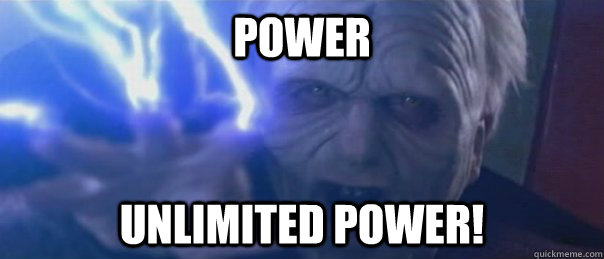# Introduction to Quantum Computing

Quantum computing is an area focused on developing computers based on quantum theory. Currently quantum computers are at a stage where classical computers stood in the 20th century. Quantum Computers have the potential to solve large problems that would take years to solve using the fastest supercomputer that exists today. Quantum Computers use quantum mechanical phenomena such as superposition and quantum entanglement to perform computation

Classical computing relies on boolean algebra, the data must be processed in either one of the binary states 1 or 0. The transistors and microprocessor are becoming smaller and smaller everyday and there is a limit to how much we can reduce it's size, as this limit reaches the classical laws fail to be applied and the quantum world takes over. In quantum computing the bits are referred as a qubit or a quantum bit. If we imagine a qubit to be an electron in a magnetic field, the electron having two spin states( +/- 1/2 ) the spin up and spin down states can be considered as on and off states. Now here come the twist what if I told you the electron can be in a state of spin which is a combination of the two spin states.This is one of the basic concepts in quantum computing.

Superposition

The system can be in a state where it is either in some particular state or a combination of the state with each state having some probability of existence. If we consider a two state system say a qubit, the qubit can either be on(1) or off(0) or the qubit can exist in a state of a|1> + b|0>  where a² and b² are the probabilities of the state being 1 or 0, and a²+b²= 1.

Now lets say we have a 20 qubit register and when the qubit enters into superposition it can have the power to do 220 calculations in a single step. That's the beauty of Quantum ComputationNow a question comes, how do all these qubits in a register interact with each other? Quantum Entanglement to the rescue

Quantum Entanglement

Quantum Entanglement is one of the strangest and spookiest aspect in quantum physics. Particles like electrons and photos exhibit a property where the state of one of the particle can be described with reference to the other particle, even when both the particles are separated by a huge distance, the particles are said to be entangled. Assume two electrons are entangled and these electrons are put into superposition. and we measure one of the particles( measurement always causes the superposition to collapse into a particular state ) say that the measurement caused the particle to collapse into a 1 state when we measure the other particle when measured will always reuslt in say a 0 state and this will continue as long as the particles are entangled. Einstein called it "spooky action at a distance"

Together superposition and entanglement lays the foundation of quantum computing. In classical computing a 2 bit register can only store one of the four values while a 2 qubit register can store all four numbers simultaneously and when more qubits are added the computational power increases exponentially.

The Future is Quantum

July 1, 2019, 10:41 p.m.